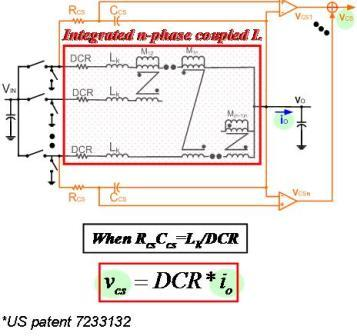LIBRARY

# DCR current sensing for coupled inductor VR (2005)Current sensing is necessary for microprocessor VRs for the Adaptive Voltage Positioning (AVP), current sharing and current protection. In today microprocessor VR, DCR current sensing method is most popular one. However, the normal DCR current sensing method is not valid for coupled inductor VRs for two reasons: Firstly, the inductance which is needed for the time constant matching in the normal DCR current sensing method become fuzzy in coupled inductor VRs since there are several inductances(Lself, Lk, Lss, Ltr, etc.) in coupled inductor VRs. Secondly, the phase current waveform is different from the normal DCR current sensing waveform.

To solve this issue, the DCR current sensing method for coupled inductor VR is proposed. After derivation, it is found that when the time constant of the current sensing network matches the time constant of the leakage inductance and DCR of the coupled inductor, the DCR current sensing network can sense the total inductor current instantaneously.

Therefore, we can use this DCR current sensing method to do the total current protection. Further analysis also shows that the AVP function and the current sharing function can be achieved with this DCR current sensing method.

The following figure shows the coupled inductor VR DCR current sensing method diagram.